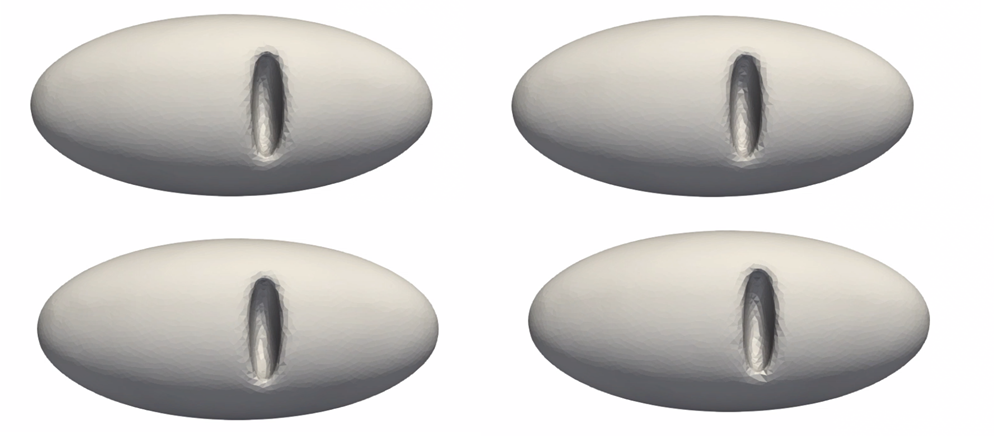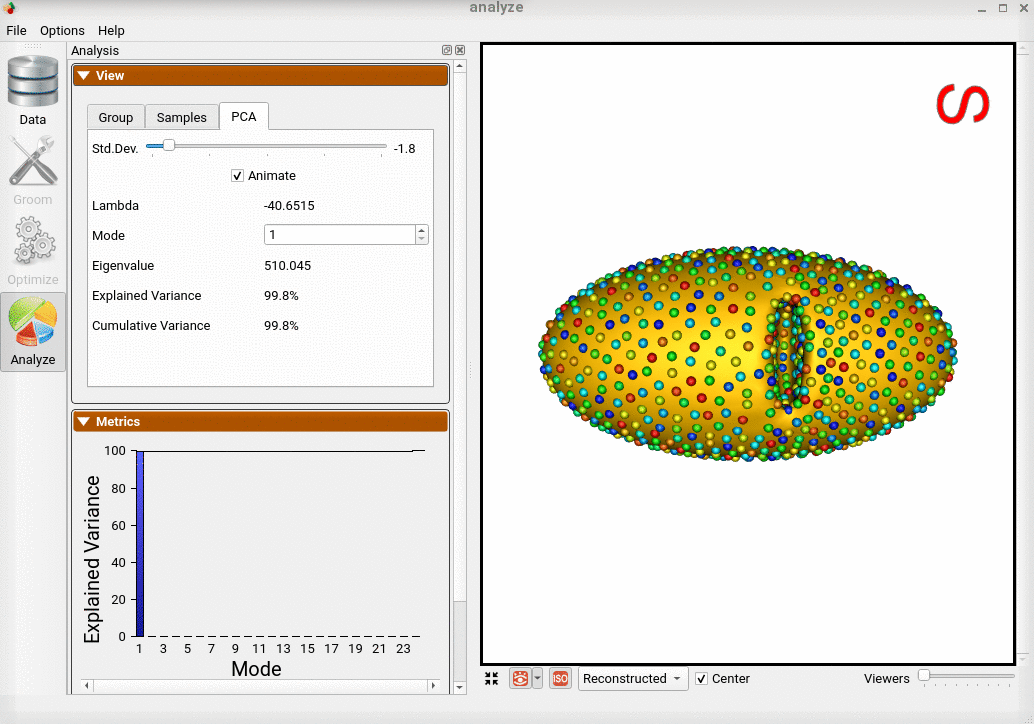# Thin Cavity Bean: Shape Model with Geodesic Distances

## What and Where is the Use Case?

This use case demonstrates using ShapeWorks tools to perform the following.

• Geodesic distance based repulsion for mesh domains
• Build a shape model where shapes are given as triangular surface meshes

The use case is located at: Examples/Python/thin_cavity_bean.py

## Running the Use Case

To run the use case, run RunUseCase.py (in Examples/Python/).

$cd /path/to/shapeworks/Examples/Python$ python RunUseCase.py thin_cavity_bean


This calls thin_cavity_bean.py (in Examples/Python/) to perform the following.

• Loads the thin_cavity_bean dataset using a local version if it exists (i.e., previously downloaded); otherwise, the dataset is automatically downloaded from the ShapeWorks Data Portal.
• Optimizes particle distribution (i.e., the shape/correspondence model) by calling optimization functions in OptimizeUtils.py (in Examples/Python/). See Optimizing Shape Model for details about algorithmic parameters for optimizing the shape model.
• Launches ShapeWorks Studio to visualize the use case results (i.e., the optimized shape model and the groomed data) by calling functions in AnalyzeUtils.py (in Examples/Python/).

## Grooming Data

In this use case, we download pre-groomed data. The use case will be updated soon to demonstrate the full mesh grooming process.

Here is an example of the data:## Optimizing Shape Model

Below are the default optimization parameters when running this use case. For a description of the optimize tool and its algorithmic parameters, see: How to Optimize Your Shape Model.

{
"number_of_particles": 1024,
"use_normals": 1,
"normal_weight": 10.0,
"checkpointing_interval": 200,
"keep_checkpoints": 0,
"iterations_per_split": 150,
"optimization_iterations": 2500,
"starting_regularization": 3000,
"ending_regularization": 0.1,
"recompute_regularization_interval": 1,
"domains_per_shape": 1,
"domain_type": 'mesh',
"relative_weighting": 15,
"initial_relative_weighting": 0.01,
"procrustes_interval": 0,
"procrustes_scaling": 0,
"save_init_splits": 0,
"verbosity": 3,
"geodesics_enabled": 1,
}


## Analyzing Shape Model

ShapeWorks Studio visualizes/analyzes the optimized particle-based shape model by visualizing the mean shape, individual shape samples, and the shape modes of variations. For more information, see: How to Analyze Your Shape Model?.